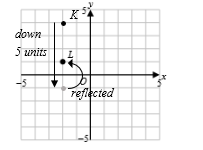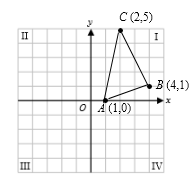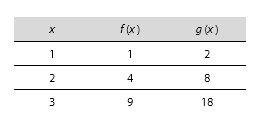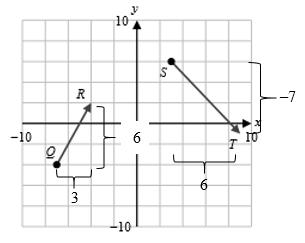## 1.

The Correct Answer is (D) — You are given a point resulting from a few translations; to find out where the original point was located, simply apply the reverse of those translations, working backwards from the final point. K was translated down 5 units and then reflected across the x axis to achieve L at (–2,1). Reflecting L across the x axis gives you (–2,–1). Since K was translated down 5 units, this new point must be translated up 5 units! K is therefore (–2,4). If this is hard for you to visualize, you can always trace it out on the graph:## 2.

The Correct Answer is (F) — To answer this question, you can simply plug the two points, B (7,3) and F (2,10), into the distance formula:

## 3.

The Correct Answer is (A) — You could use the rise-over-run slope formula , plugging in two points you can read off the graph, but a much quicker method is to eyeball it when possible. For every unit decrease in the y direction, there is an increase by 3 units in the x direction. Following from the definition of slope as rise over run, this means the slope would be equal to —.

If you chose (D), you probably did run over rise, putting the change in the x direction over the change in the y direction instead.

If you chose (E), you probably forgot that the line is decreasing.

If you chose (B) or (C), you probably misread the graph.

## 4.

The Correct Answer is (J) — This question gives you an easy-to-read graph; notice that the y-intercept of the line occurs at y = 3. In y-intercept form, y = mx + b. The y-intercept is represented by b. The only answer choice that has a b value of 3 is (J).

If you chose (G), you probably found the slope of the line correctly but forgot that it was shifted upwards.

## 5.

The Correct Answer is (D) — The question asks you to find the two points that produce a slope with an absolute value greater than 5. You can eliminate all answer choices where the absolute value of the difference between y values, the rise, is less than 5. This is because all of the x values will produce whole number differences that will decrease the magnitude of the slope.

For example, (6,3) and (4,2) have a slope of , eliminating (B). Similarly, the rise for (0,2) and (4,2) is 0, for (0,0) and (3,4) is —4, and for (–6,7) and (–4,8) is –1. Without even calculating the run, you can eliminate (A), (C), and (E).

This leaves you with (D), (–2,4) and (–1,10), which has a slope of .

## 6.

The Correct Answer is (H) — To solve this question, find the area of the part of the square in quadrant II and divide by the total area of the square. The vertex of the square in this quadrant is (–3,1), and you know it will intersect with the y- and x-axis at (0,1) and (–3,0) respectively; it forms a rectangle with side lengths of 1 and 3, with an area of 1 × 3 = 3. The side length of the square is the distance between any two adjacent vertices, picking (1,1) and (–3,1), you can find this distance to be 1 – (–3) = 4, so the total area of the square is 4 × 4 = 16. Therefore, the percentage of the square that lies in quadrant II is .

## 7.

The Correct Answer is (D) — If line b is parallel to line l, they will have the same slope. The slope of line l is equal to , which you can read off the graph without using points to calculate it, as . If you got (A), you probably thought the line was decreasing.

If you got (B), you probably thought b was perpendicular to l, in which case its slope would be the negative reciprocal of l.

## 8.

The Correct Answer is (J) — Line k is perpendicular to line l, so its slope will be the negative reciprocal of the slope of line l. The slope of line l is equal to , which you can read off of the graph as . Therefore, the slope of line k is . You know that k has a y-intercept of 2, so its equation in y-intercept form is .

If you chose (G), you probably thought that line k was parallel to line l, and that its slope was the same.

If you chose (F) or (K), you may have thought that k had the same y-intercept as l.

## 9.

The Correct Answer is (B) — For questions that ask you to compare the slopes of more than two lines, it may be faster to sketch a graph first:Points A and C have the largest vertical distance between them, as well as the smallest horizontal distance. Therefore, you can see that the steepest slope is the line that connects A and C, or .

## 10.

The Correct Answer is (G) — In linear equations of the form y = mx + b, m is the slope. The first line has the equation , so you know that its slope is . It is perpendicular to the second line given to you, so that means their slopes are negative reciprocals of each other. Therefore, the value of m is –2.

If you chose (J), you probably thought that the two lines were parallel, meaning their slopes would be equivalent.

## 11.

The Correct Answer is (A) — This question is asking you to compare vertical stretches of a parabola of the form p (x) = a(xb)2, where a is the variable controlling the transformation. Recall that, for values of a where its magnitude is greater than 1, the parabola looks like it is being stretched, and becomes “skinnier”. You can prove this by comparing values of and :For each value of x, the function with the larger a value produces a greater y value. Similarly, you can compare outputs along the same x value for the three graphs to see whose y values, and thus a values, are the greatest, to find that F > G > H.

## 12.

The Correct Answer is (G) — Since you know the slope of line b, you can partially construct its equation in y-intercept form, y = mx + c, where m is the slope and c is the y-intercept: y = 7x + c. Substituting in the given point, (2,3), you can solve for the y-intercept:

## 13.

The Correct Answer is (D) — Another way of expressing the component form of vectors is by using the unit vectors, i and j. For example, q = can also be written as q = 2i + 4j, where i = and j = . You can treat i and j as regular variables, allowing you to solve the equation for s:

Rewriting the expression for s, you can find its component form to be equal to .

## 14.

The Correct Answer is (G) — The slope of the line shown in the figure is 2, so the slope of the line perpendicular to it will be .

A and B form a line with a positive slope, so you can eliminate (F). C and D form a line with a slope of infinity, so (H) is out. D and F form another line with a positive slope, so you can eliminate (J). E and F look tempting because the line has a negative slope, however it decreases at a rate of 4 units for every unit increase in the x direction, i.e. it has a slope of –4, which is too steep, so you can also eliminate (K).

The only option left is (H), and indeed, B and C create a line segment with a slope of exactly .

## 15.

The Correct Answer is (C) — Recall that the formula for the equation of a circle in the standard (x,y) coordinate plane is given by (xh)2 + (yk)2 = r2, where the vertex is (h,k) and r is the radius. A circle with an equation (x − 4)2 + (y + 2)2 = 25 thus has a vertex of (4,−2), meaning it would be 4 units right and 2 units down from a circle originally located at the origin.

If you chose (B), you probably missed that the positive sign in front of the 2 actually means down instead of up.

## 16.

The Correct Answer is (K) — This question asks you to analyze the effect of various translations on a parabola. Recall that, given f (x) = (x + a)2 + b, a corresponds to a shift in the negative x direction and b corresponds to a shift in the positive y direction. Since both a and b are positive integers, the resulting parabola should be up and to the left of the origin.

If you chose (H), you forgot that a positive value for a actually means a shift to the left.

## 17.

The Correct Answer is (D) — To find the unit vector notation, ai + bj, of each of the individual vectors, remember that the coefficient in front of i is equal to its length in the positive x direction, and the coefficient in front of j is equal to its length in the positive y direction. You can use the graph given to you to count the length in both directions. Make sure to read the scale of the axes carefully, and to count from tail to tip:The unit vector notation of is 3i + 6j, and the notation of is 6i – 7j (minus 7 because it is pointing downwards). Adding them together is only a matter of collecting like terms, and treating i and j like separate variables:

+ = (3i + 6j) + (6i – 7j) = 9ij

If you chose (C), you probably subtracted from .

## 18.

The Correct Answer is (G) — You should be able to solve this question without any tedious calculations. First, notice that the steeper slope of the first line segment means the particle is moving quicker in the first section of time than it is during its last section. Also, you can see that it is moving at 1 m/s for this first section, as the slope is
.

Already, you can eliminate answer choices (J) and (K), because they say that at first the particle is moving at 4 m/s, or II. Since you know that the particle is moving slower in the last section, you can eliminate (F) and (H), because they both describe a speed greater than 1 m/s. You are left with (G), which you can double check easily: the particle’s initial speed is 1 m/s, it does not move for a while (0 m/s), and finally it moves at a speed of m/s.